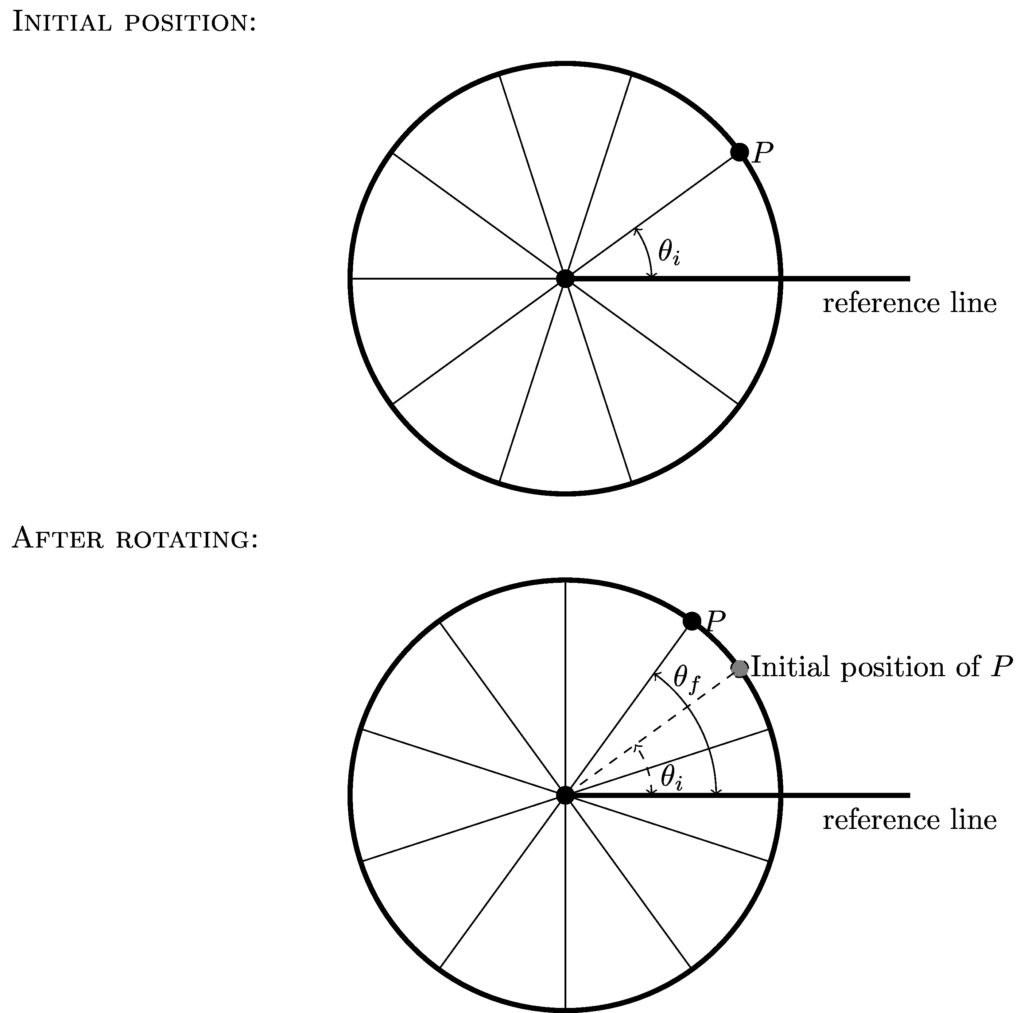# Chapter 5: Rotational motion

## 5.1 Angular position, velocity, and acceleration

When we described the motion of an object moving in a straight line, we defined a coordinate system to refer to. We used the Cartesian system for translational motion. If you want to describe the motion of a rotating object, we’ll define angular quantities that are analogous to the translational position, velocity and acceleration that we have come to know and love.

### 5.1.1 Angular position,$$\theta$$

First, look at the rotating object and determine which point it is rotating around—the axis of rotation is the point that all parts of the object rotate in a circle around. Now, draw a line out from the axis of rotation; this is our reference line, from which we will measure all angles. Just like when we were dealing with the Cartesian grid, our reference line may be going in any arbitrary direction, as long as we are consistent through an entire problem.

As a point travels around the axis of rotation, it “sweeps out” an angle. If you draw a line from the axis to the point in question, there will be some angle between that line and the reference line. This is the point’s angular position, and it labeled with the Greek character $$\theta$$. See the figure below for an illustration.

$\theta = \textrm{angle measured from reference line} \tag{5.1}$As the wheel rotates, point $$P$$ moves from one location to another. We can define that motion in terms of the angle it has rotated through. Note that the reference line is not part of the wheel, and does not move.

The unit for angular position is the radian (rad). There are $$2\pi$$ radians in a complete circle.

But direction is also important, right? For direction, we use the same conventions as before: positive angles are measured counterclockwise (known in other English-speaking cultures as “anticlockwise” or “widdershins”) from the reference line.

A radian is the angle for which the arc length on a circle of radius r is equal to the radius of the circle; see the figure below.When the arc length ($$s$$) of a circular segment is the same length as the radius ($$r$$), the angle ($$\theta$$) is one radian.

This unit defines an angular measure that is relative to the circle itself, and this makes it very useful in rotational motion calculations. For example, you’ll recall from geometry that the arc length ($$s$$) corresponding to a particular angle ($$\theta$$) measured in radians is

$s = r\theta \tag{5.2}$

A similar relationship is not available if you are measuring in degrees. Note that both $$s$$ and $$r$$ have dimensions of length. The only way for this expression to be dimensionally correct is if radians are dimensionless; this means you can effectively ignore radians when performing unit analysis.

#### Example 5.1

Given the circumference of a circle is $$C = 2\pi r$$, how many radians are in half a circle?

The arc length around half of a circle is the half the circumference:

\begin{align*} s &= r\theta \\ \frac{2\pi r}{2} &= r\theta \\ \theta &=\pi \end{align*}

There are $$\pi$$ radians in half a circle (180°).

### 5.1.2 Angular velocity, $$\omega$$

As an object rotates, a point on the object changes its angular position. Given the time $$\Delta t$$ it takes to make this change, we have the angular velocity:

$\omega = \frac{\Delta\theta}{\Delta t} = \frac{\theta_f – \theta_i}{\Delta t} \tag{5.3}$

The unit of angular velocity is radians per second (rad/s). The symbol $$\omega$$ is a lowercase Greek letter omega. The sign convention for angular velocity is the same as for angular position. A positive angular velocity represents counterclockwise motion.

### 5.1.3 Angular acceleration, $$\alpha$$

So what if the angular velocity is changing? Then we have angular acceleration:

$\alpha = \frac{\Delta\omega}{\Delta t} \tag{5.4}$

The units of angular acceleration is radians per second per second (rad/s/s), usually simply referred to as radians per second squared (rad/s2). The symbol $$\alpha$$ is the lowercase Greek letter alpha.

The direction of angular acceleration is given by the sign of the change of the angular velocity. If the final angular velocity is smaller than the initial, then the angular acceleration is negative. Note that the direction of rotation is not particularly importantonly how the angular velocity is changing is what matters. This is similar to translational acceleration as studied in chapter 4, and the table in section 4.4 comparing speed to directions of velocity and acceleration applies just as well to angular motion.